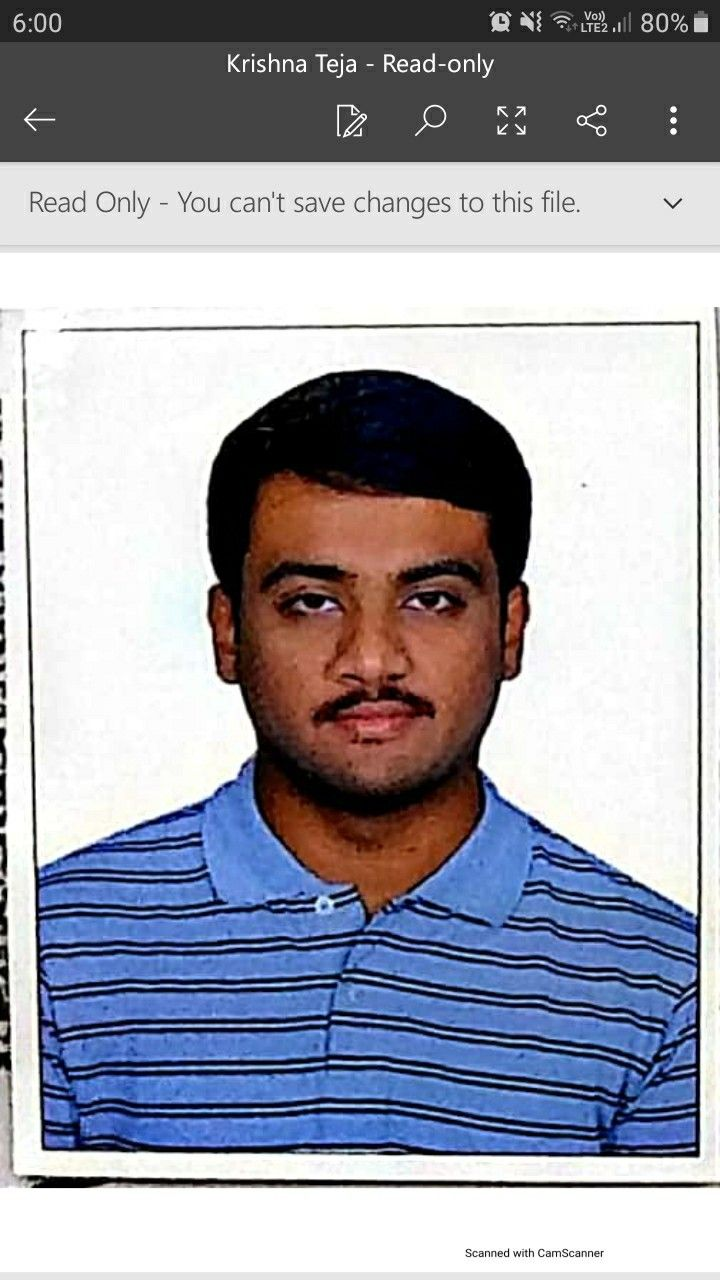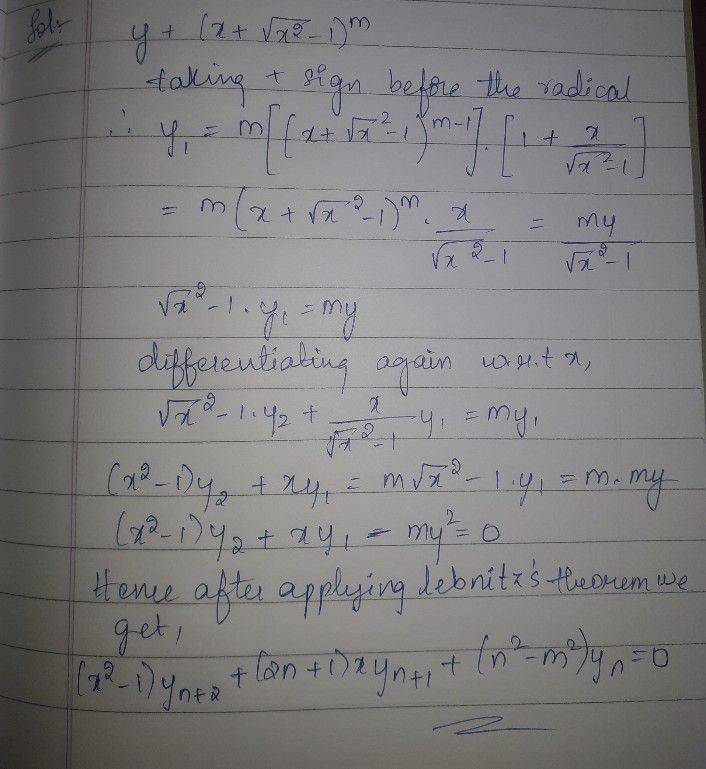Symbol
Problem$120$ $\left(1\right)$ $Ify=log$ $\left(x+\sqrt{1+x^{2}} \right)^{2}$ $1$ prove that $\left(1+x^{2}\right)y_{n+2}+\left(2n+1\right)xy_{n+1}+n^{2}y_{n}=0$ $1$ $2$
Calculus
Search count: 105
SolutionQanda teacher - krishna